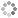# PROWAREtecharticles » current » dot-net » common-activation-functions

## .NET: Common Activation Functions with Their Derivatives in C#

The neural network activation functions Rectified Linear Unit, Leaky Rectified Linear Unit, Exponential Linear Unit, Hyperbolic Tangent, Sigmoid and the derivates for each.

Leaky ReLU and ELU adress the "dying ReLU" problem which comes from setting values to zero. Both are recommended over the original ReLU procedure. Sigmoid is still used for binary output neural networks For some networks, Sigmoid and tanh should suffice.

``````
public static class ActivationFunctionsWithDerivatives
{
public static double ReLU(double x) // Rectified Linear Unit function
{
return x > 0 ? x : 0;
}

public static double ReLUPrime(double x) // derivative of ReLU
{
return x > 0 ? 1 : 0;
}

public static double Sigmoid(double x) // and oldie but goodie, ReLU has replaced it for the most part
{
return 1.0 / (1 + Math.Exp(-x));
}

public static double SigmoidPrime(double x) // derivative of Sigmoid
{
return (1.0 / (1 + Math.Exp(-x))) * (1.0 - (1.0 / (1 + Math.Exp(-x)))); // this is simply: Sigmoid(x) * (1.0 - Sigmoid(x))
}

const double alpha = 0.01; // default is 0.01, but this can be modified, bigger or smaller

public static double LeakyReLU(double x) // Rectified Linear Unit function (Leaky variant, more modern than ReLU)
{
return x >= 0 ? x : (alpha * x);
}

public static double LeakyReLUPrime(double x) // derivative of Leaky ReLU
{
return x >= 0 ? 1 : alpha;
}

public static double ELU(double x) // Exponential Linear Unit function
{
return x >= 0 ? x : (alpha * (Math.Exp(x) - 1));
}

public static double ELUPrime(double x) // derivative of ELU
{
return x >= 0 ? 1 : (alpha * Math.Exp(x));
}
public static double Tanh(double x) // Hyperbolic Tangent function
{
return (Math.Exp(x) - Math.Exp(-x)) / (Math.Exp(x) + Math.Exp(-x));
}
public static double TanhPrime(double x) // derivative of Tanh
{
return 1 - ((Math.Exp(x) - Math.Exp(-x)) / (Math.Exp(x) + Math.Exp(-x))) * ((Math.Exp(x) - Math.Exp(-x)) / (Math.Exp(x) + Math.Exp(-x))); // this is simply: 1 - (tanh(x) * tanh(x))
}
}
``````This site uses cookies. Cookies are simple text files stored on the user's computer. They are used for adding features and security to this site. Read the privacy policy.